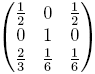# Undercurrents

In this episode, the FBI is searching for a group of girls from China that was brought into the country illegally. The girls might be infected with bird flu, which could cause a pandemic of great proportions.

Many mathematical concepts are used in this episode. We are going to focus on how to model disease dynamics, Ceasar ciphers, and probability matrices.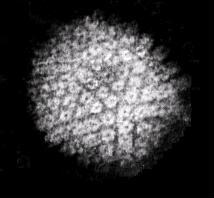Mathematicians have modeled the spread of diseases and other epidemiologic phenomena. Usually compartmental models are used to describe an epidemic. These consist of separating a population into disjoint classes, which are represented by compartments. There are also arrows between the classes indicating the movement of people from one class to another.

### SIR model

SIR is a very common compartmental model. It consists of three classes: S (stands for susceptible), I (stands for infectious), and R (stands for recovered). A susceptible person is someone who is not infected, an infectious person is someone who is infected and is able to infect other people, and a recovered person is no longer infectious. The picture below describes the SIR model. We assume that people are born susceptible to a disease (say the flu), and some become infectious and then recover.In most epidemics, people acquire diseases due to direct contact with infectious people. Here is a link to a Java Applet that models the spread of a disease .

Activity 1

For the Herpes Simplex Virus type 1 (HSV-1 or oral herpes), infections occur as follows: A person obtains the disease after direct contact with someone who is infectious. Once a person gets HSV-1, the person will always have the disease. An infected person may or may not show symptoms, but will have recurrent periods of being infectious (able to infect someone else) until death. Write a compartmental model that describes an HSV-1 infection in a population.

## Caesar Shift

When Charlie and Larry were trying to figure out the message on the woman's foot, they were able to convert the I Ching characters into digits. Then they tried to understand the meaning of the digits using Caesar Shift, a very simple cryptosystem.

For convenience, one usually uses numbers from 0 to N-1 to represent an alphabet of N characters. Suppose we are using the regular 26-character alphabet A, B, C, ..., Z. Then we encode the characters using the table below.

 0 1 2 3 4 5 6 7 8 9 10 11 12 13 14 15 16 17 18 19 20 21 22 23 24 25 A B C D E F G H I J K L M N O P Q R S T U V W X Y Z

The idea behind the Caesar Shift is a function from the set {0,1,2,...,25} onto itself given by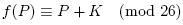where P stands for any digit from 0,1,..., 25. The operation is performed in modular arithmetic; that is if P+K is not in the range 0, ..., 25, we have to add or subtract 26 enough times to get the result in that range. For instance if K = 3 the above function would yield the following table:

 0 1 2 3 4 5 6 7 8 9 10 11 12 13 14 15 16 17 18 19 20 21 22 23 24 25 D E F G H I J K L M N O P Q R S T U V W X Y Z A B C

The function f is called the shift, and K is referred to as the key. The comparison of the two tables explains the shift.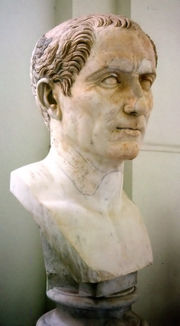The Caeser shift is named after Julius Caesar (100 BC - 44 BC), who used the cipher to send coded military messages to his generals.
Activity 2
1. Create your own code by giving different values to K. Notice that K can be negative as well. (The sign of K determines if the code is a left or right shift).

2. Suppose you are given a text that has been encoded using a Caesar shift. If you know K, how can you decode the message?

3. Do you think that giving higher values of K make the code harder to break?

### Breaking the Caesar shift

Clearly, if we knew the value of K, we know how to decode a message. However, if a message is not intended to for you, you won't know the value of K. In this case, there is a method called frequency analysis. This method exploits the fact that some characters occur more often than others. For example, in English the letter "E" is the most common letter. To use frequency analysis to break a Caesar cipher, look at an encoded message and find the most repeated character. Then find K, assuming that this character is the most frequent one used in the language (i.e. "E"). Of course this method works better if one has a very long message. For shorter ones, there is the "trial-and-error" approach, which consists of simply trying all the possible keys (in English there are 26 of them).

Activity 3
1. Using frequency analysis, decode the following message that has been encrypted using Caesar shift:
PXPXKXENVDRUXVTNLXHYMXGMAXYKXJN
XGVRFXMAHWGXXWLEHGZXKVBIAXKMXQM

2. Decode the message UFDRJSTB, if you know that it has been encoded using Ceasar shift.

Although the Caesar cipher is not considered secure today, it was probably quite effective during Caesar's reign. In fact, most of Caesar's enemies didn't even know how to read, much less decipher his coded messages. Frequency analysis wasn't developed until nearly 1,000 years later, by an Arab mathematician named Al-Kindi.
As you have noticed, this cryptosystem is too simple to be very secure. That's why later someone came up with a generalization of the Caesar shift function called an affine map. In this cipher, letters are coded according to the following function: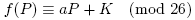where a is a fixed integer. This system is more secure because in order to decrypt a message, the receiver must know both K and a.

Activity 4
In the 27-character alphabet (where blank=26), use the affine cipher with a=13 and K=9 to encode the message "HELP ME".

## Probability Matrix

At some point during the episode, Charlie and Larry used a probability matrix to find the location of the missing girls. We now discuss what a probability matrix is. Suppose we have a sequence of experiments with possible outcomes a1, a2, ..., an. We further assume that the outcome ai on any given experiment depends only on the outcome of the immediately proceeding experiment (not any of the other experiments). This is called a Markov chain process.

Markov processes are often used to model events in the real world. For example, suppose that an amateur meteorologist notices that the weather on a given day seems to depend on the weather conditions of the previous day. She observes that if it is raining one day, then the next day is sunny 60% of the time and rainy 40% of the time; on the other hand if it is sunny, the next day is sunny with probability 30% and rainy with probability 70%. In this Markov process, there are two outcomes (sunny and rainy). The transition probability between sunny and rainy is 70%, between sunny and sunny is 30%, between rainy and sunny is 60%, and between rainy and rainy is 40%.

More generally, we let pij be the transition probability between outcomes ai and aj. These probabilities can be represented compactly in a probability matrix (also known as a transition matrix or a stochastic matrix) like this: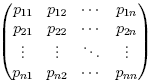Markov processes can be drawn as a transition diagram. In a transition diagram, each outcome (or state) is drawn as a vertex. Arrows are shown to indicate possible transitions between states, and the numbers on each arrow indicates the transition probability. For instance, the simple weather forecasting Markov process that we described above can be represented as: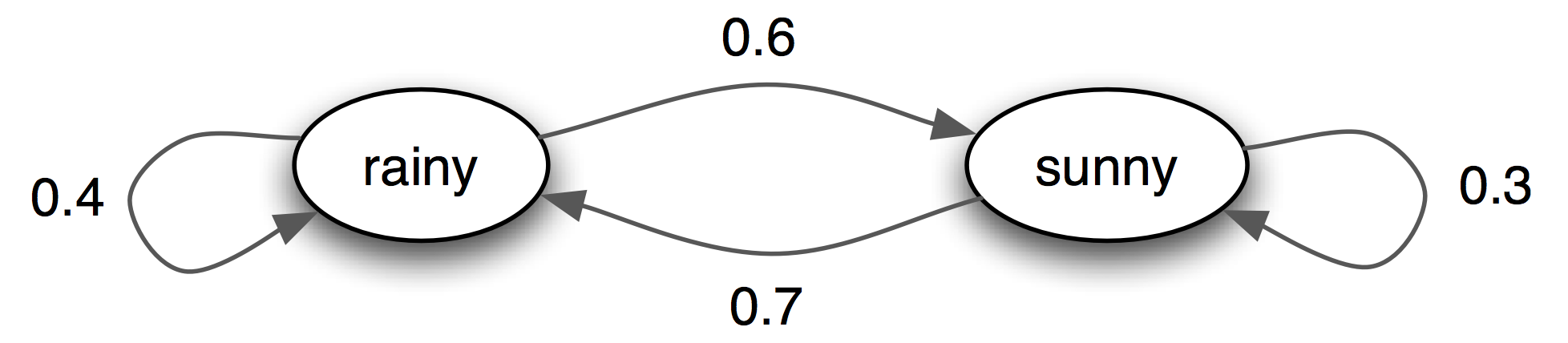Activity 5: Obtaining probability matrix from transition diagram

1. For a Markov chain process with outcomes a1,a2,...,an, what is the value of pi1+pi2+...+pin?
2. Consider the following transition diagram: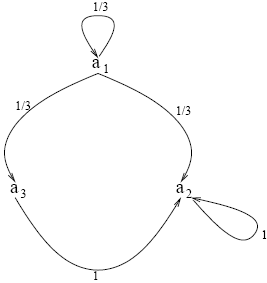Draw the corresponding probability matrix.
3. Draw the transition diagram for the probability matrix below